### Superscripts and Subscripts

Subscripts and superscripts are obtained using the special characters `_` and `^` respectively. Thus the identity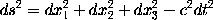is obtained by typing
```\[ ds^2 = dx_1^2 + dx_2^2 + dx_3^2 - c^2 dt^2 \]
```
It can also be obtained by typing
```\[ ds^2 = dx^2_1 + dx^2_2 + dx^2_3 - c^2 dt^2 \]
```
since, when a superscript is to appear above a subscript, it is immaterial whether the superscript or subscript is the first to be specified.

Where more than one character occurs in a superscript or subscript, the characters involved should be enclosed in braces. For example, the polynomial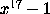is obtained by typing `\$x^{17} - 1\$`.

One may not type expressions such as `\$s^n^j\$` since this is ambiguous and could be interpreted either as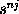or as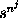. The first of these alternatives is obtained by typing `\$s^{n j}\$`, the second by typing `\$s^{n^j}\$`. A similar remark applies to subscripts. Note that one can obtain in this way double superscripts (where a superscript is placed on a superscript) and double subscripts.

It is sometimes necessary to obtain expressions in which the horizontal ordering of the subscripts is significant. One can use an `empty group' `{}` to separate superscripts and subscripts that must follow one another. For example, the identity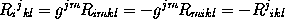can be obtained by typing
```\[ R_i{}^j{}_{kl} = g^{jm} R_{imkl}
= - g^{jm} R_{mikl} = - R^j{}_{ikl} \]
```